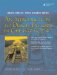# Input and Output

In Example 1.3, the directive

```#include
```

allowed us to make use of the predefined global input and output stream objects.

They are

1. cin standard input, the keyboard by default
2. cout standard output, the console screen by default
3. cerr standard error, another output stream to the console screen that flushes more often and is normally used for error messages

To send output to the screen in Example 1.3, we made use of the global stream object (of the class ostream), called cout. We called one of its member functions, whose name is operator<<(). This function overloads the insertion operator << and defines it like a global function. The syntax for that output statement is also quite interesting. Instead of using the rather bulky function notation:

 We will discuss overloaded functions and operators in more detail later in Section 5.2.

```cout.operator<<("Factorial of :");
```

we invoked the same function using the more elegant and readable infix syntax:

```cout << "Factorial of:" ;
```

This operator is predefined for use with many built-in types, as we see in the next output statement.

```cout << "The cost is \$" << 23.45 << " for " << 6 <<
"items." << '
';
```

In Example 1.4, we can see the operator>>() used for input with istream in an analogous way to << for ostream.

Example 1.4. src/iostream/io.cpp

 ```#include #include int main() { using namespace std; const int THISYEAR = 2006; string yourName; int birthYear; cout << " What is your name? " << flush; cin >> yourName; cout << "What year were you born? " ; cin >> birthYear; cout << "Your name is " << yourName << " and you are approximately " << (THISYEAR - birthYear) << " years old. " << endl; } ```

The symbols flush and endl are manipulators that were added to the std namespace for convenience. Manipulators are implicit calls to functions that can change the state of a stream object in various ways.

 We discuss these further in Section 1.10.

Notice also that we are using the string type from the Standard Library. We will discuss this type and demonstrate some of its functions shortly (see Section 1.9).

Exercises: Input and Output

1.

Using Example 1.4, do the following experiments:

• First, compile and run it to see its normal behavior.
• What happens if you enter a non-numeric value for the birth year?
• What happens if you enter a name such as Curious George as your name?
• What happens if you remove the following line?

```using namespace std;
```
• Replace the statement

```cin >> yourName;
```

with the statement

```getline(cin, yourName);
```

and try Curious George again.

• Can you explain the differences in behavior between cin >> and getline()? We discuss this later in Section 1.9.
• Add some more questions to the program that require a variety of numerical and string answers, and test the results.
2.

Consider the program shown in Example 1.5.

Example 1.5. src/early-examples/fac2.cpp

 ```#include long factorial(long n) { long ans = 1; for (long i = 2; i <= n; ++i) { ans = ans * i; if (ans < 0) { return -1; } } return ans; } int main() { using namespace std; cout << "Please enter n: " << flush; long n; <-- 1 cin >> n; <-- 2 if (n >= 0) { long nfact = factorial(n); if (nfact < 0) { cerr << "overflow error: " << n << " is too big." << endl; } else { cout << "factorial(" << n << ") = " << nfact << endl; } } else { cerr << "Undefined: " << "factorial of a negative number: " << n << endl; } return 0; } ```   (1)long int (2)read from stdin, try to convert to long
• Test the program in Example 1.5 with a variety of input values, including some non-numeric values.
• Determine the largest input value that will produce a valid output.
• Can you change the program so that it will produce valid results for larger input values?
• Modify the program so that it cannot produce invalid results.
• Explore the effects of using the statement

```if(cin >> n) { ... }
```

to enclose the processing of n in this program. In particular, try entering non-numeric data after the prompt. This is an example of the use of a conversion operator, which we discuss in more detail in Section 2.13.

• Modify the program so that it will accept values from the user until the value 9999 is entered.An Introduction to Design Patterns in C++ with Qt 4
ISBN: 0131879057
EAN: 2147483647
Year: 2004
Pages: 268